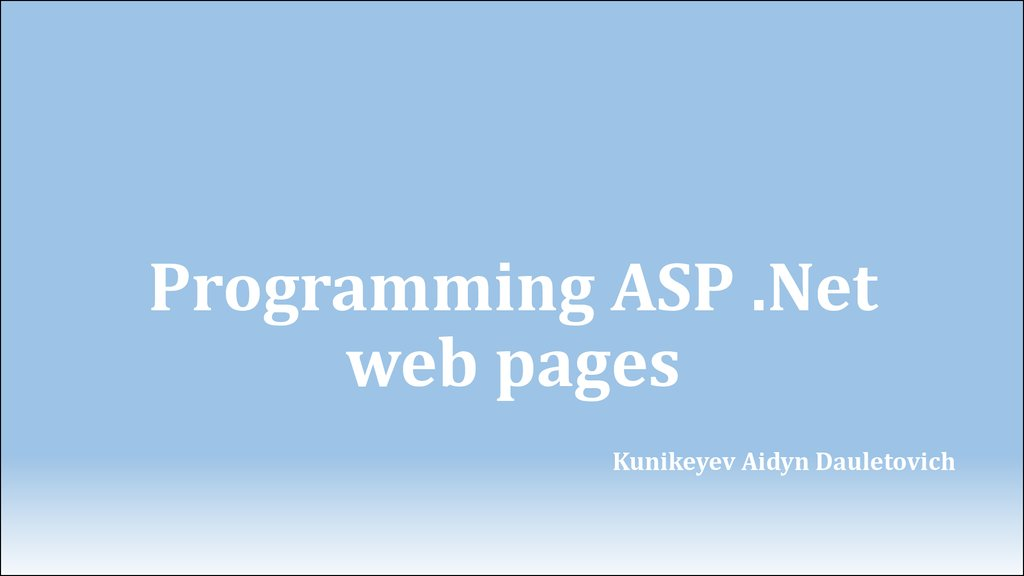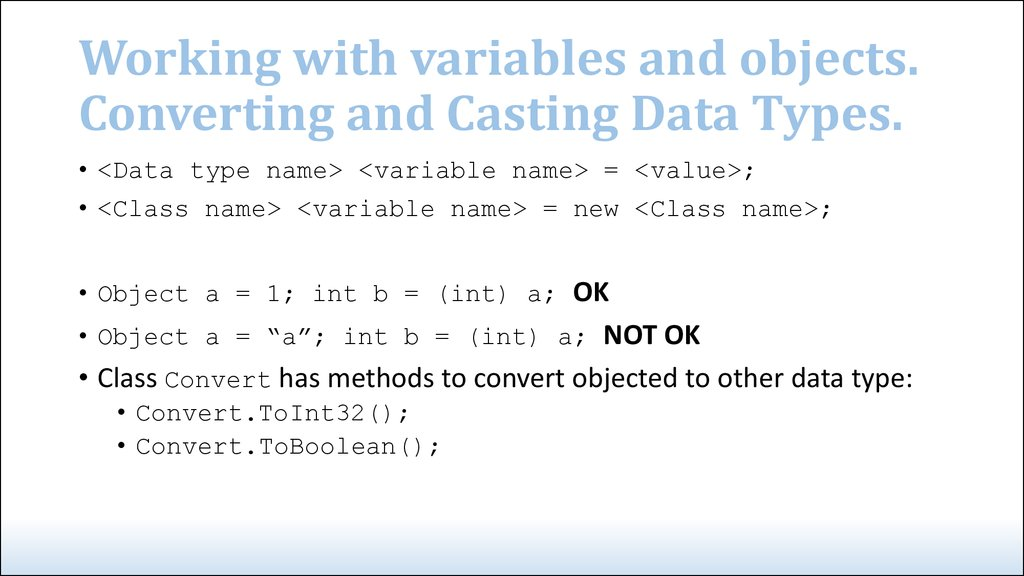# Programming ASP

## 1. Programming ASP .Net web pages

Kunikeyev Aidyn Dauletovich

## 3. Working with variables and objects. Converting and Casting Data Types.

• <Data type name> <variable name> = <value>;
• <Class name> <variable name> = new <Class name>;
• Object a = 1; int b = (int) a; OK
• Object a = “a”; int b = (int) a; NOT OK
• Class Convert has methods to convert objected to other data type:
• Convert.ToInt32();
• Convert.ToBoolean();

## 4. Using Arrays, Collections and Generics.

• string[] roles = new string;
• ArrayList roles = new
ArrayList();
• roles = "ContentManagers";
• Array.Resize(ref roles, 3);
• roles = "Members";
• List<string> roles = new
List<string>();
• List<int> intList = new
List<int>();

+=
-=
*=
/=
3;
3;
3;
3;

## 8. If and If Else Constructs

{
DeleteButton.Visible = true;
}
{
DeleteButton.Visible = true;
}
else
{
DeleteButton.Visible = false;
}

## 9. Select Case/switch Constructs

switch (today.DayOfWeek)
{
case DayOfWeek.Monday:
discountPercentage = 40;
break;
default:
discountPercentage = 0;
break;
}

## 10. Loops

for (startCondition; endCondition; step definition)
{
// Code that must be executed
for each iteration
}
while (!success)
{
success = SendEmailMessage();
}
foreach (string role in roles)
{
Label1.Text += role + "<br />";
}

## 11. Object and Constructors

public class Person
{
public Person(string firstName, string lastName, DateTime dateOfBirth)
{
_firstName = firstName;
_lastName = lastName;
_dateOfBirth = dateOfBirth;
}
}
Person myPerson = new Person("Imar", "Spaanjaars", new DateTime(1971, 8, 9));

## 12. Methods: Functions and Subroutines

// Define a function
public DataType FunctionName([parameterList])
{
return DataType
}
// Define a subroutine
public void SubName([parameterList])
{
}

## 13. Methods: Functions and Subroutines

public class Person
{
private string _firstName;
public string FirstName
{
get { return _firstName; }
set { _firstName = value; }
}
}
Person myPerson = new Person();
myPerson.FirstName = "imar";
If you want to make read-only field: just include get
If you want to make write-only field: just include set

## 14. Events

protected void Button1_Click(object sender, EventArgs e)
{
}

## 15. The end

• Laboratory work #3
• All tasks on chapter #5, Imar Spaanjaars